HSPT Math : How to do work problems

Example Questions

← Previous 1

Example Question #1 : How To Do Work Problems

Steve can paint his greenhouse in four hours working alone; his ten-year-old son can paint the greenhouse alone in nine hours. Working together, how long, to the nearest minute, would it take them?

Two hours and forty-six minutes

Two hours

Three hours and twenty-six minutes

Three hours

Three hours and six minutes

Two hours and forty-six minutes

Explanation:

Think of these work rates as "greenhouses per hour", not "hours per greenhouse". Then Steve's work rate is one fourth of a greenhouse per hour, and his son's is one ninth of a greenhouse per hour. Now, multiply each rate by the timeto get the portion of the greenhouse each paints, and add the products up to get one greenhouse. The work equation becomes: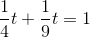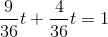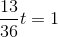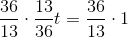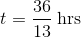or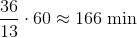This is two hours and forty-six minutes.

Example Question #2 : Word Problems

John and Tom are farmers. John can produce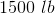of an organic product in six months. Tom can produce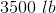of the organic product in one year. How many pounds of organic product can they produce in three years together? (Assume that they can work and produce the organic product all year round.)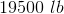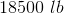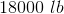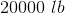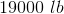Explanation:

John can producein six month. Therefore we can set up a proportion: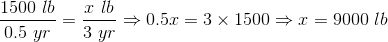Tom can producein one year. Then we can set up another proportion: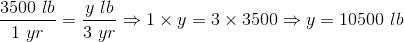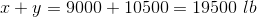Example Question #3 : Word Problems

Tom works in a mall and sells shoes. His monthly salary is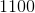dollars plus a commission ofdollars for every pair of shoes that he sells. How many pairs of shoes must he sell to earn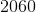dollars per month.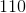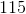Explanation:

Let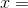number of pairs of shoes.

Then we can set up the following equation: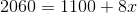Now we solve the equation for: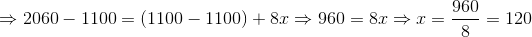Example Question #1 : How To Do Work Problems

John sells cloths. His monthly salary is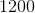dollars plus a commission ofdollars for each cloth sold. If he sellscloths this month, how much money does he make in dollars?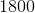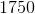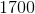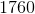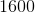Explanation:

In order to find how much he makes from selling the cloths, we first multiply the number of cloths sold bydollars.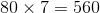dollars

Now we add his monthly salary: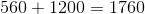dollars

Example Question #1 : Word Problems

Machine A can produce 4 books in 2 days. Machine B can produce 20 books in 4 days. How many books can machines A and B, working together, produce in 40 days?

200

280

120

960

10

280

Explanation:

Through simplification, we can see that machine A produces 2 books a day and that machine B produces 5 books a day. If we multily each by 40 days and add them together, we find that together they can produce 280 books in 40 days: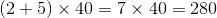Example Question #2 : How To Do Work Problems

Julie sells cars. She has a monthly salary of $1000 and a commission of$500 for each car sold. How much money will she make this month if she sells 7 cars?

$7000$1500

$4500$8000

$3500 Correct answer:$4500

Explanation:

To find out how much Julie makes from selling the cars, we first multiply the 7 cars she sells by the $500 that she receives for each car. This gives us$3,500. We now add the $1,000 that she gets for her monthly salary for a total of$4,500.

Example Question #7 : Word Problems

Bob gets paid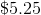an hour for the regular hours he works and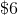an hour for any overtime hours he works. All hours over 40 in a week are considered overtime. If Bob works 44 hours this week, how much did he make?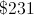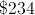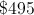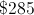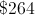Explanation:

You first calculate how much he makes for normal hours, which is the number of hours works multiplied by the wage. So for normal hours,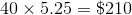. For overtime, it is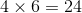. Add the amount made in overtime and the normal hours and you get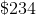.

Example Question #8 : Word Problems

A bakery has a machine that can make two hundred loaves of bread in one hour, and a machine that can make three hundred loaves of bread in one hour. How many hours will it take for the two machines together to make 10,000 loaves of bread?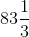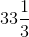Explanation:

In one hour, the two machines can make a total of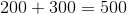Divide 10,000 loaves by 500 loaves per hour: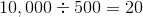.

Example Question #3 : Word Problems

A candymaker has two machines. One can turn out one hundred pieces of divinity in one hour; the other, an older machine, can do the same in one hour and twenty minutes. Working together and starting at the same time, how many hours would it take for them to make 10,000 pieces of divinity?Explanation:

The newer machine can make 100 pieces of divinity per hour. The older machine makes 100 pieces in one hour twenty minutes, or, equivalently, one and one third hours, which is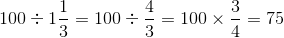pieces per hour.

Together, the machines make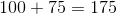pieces per hour.

Divide 10,000 pieces by 175 pieces per hour: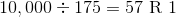.

The candy can be made in a little over 57 hours. The closest choice is therefore 60 hours.

Example Question #4 : Word Problems

A candymaker specializes in making "turtles" (pecan caramel candies). She has two machines - a newer machine, which can make one hundred turtles in seventy minutes, and an older machine, which can make one hundred turtles in ninety minutes.

She wants to make one hundred boxes of turtles, each of which holds forty turtles. How many hours would it take for both machines, working together, to produce enough turtles to fill those boxes?Explanation:

The newer machine makes one hundred turtles in seventy minutes, or, on the average,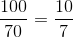turtles in one minute.

Similarly, the older machine makes, on the average,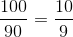turtles in one minute.

Together, they make an average of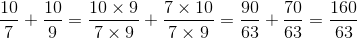turtles per minute.

The candymaker wants one hundred boxes, each with forty turtles, so she wants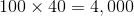turtles. Divide this by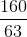turtles per minute to get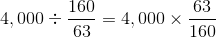minutes;

multiply this byto get the number of hours: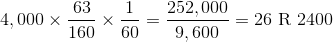The process takes just over 26 hours; the response closest to the correct time is 25 hours.

← Previous 1